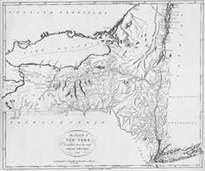# Upstate Number Theory

#### April 29 – May 1, 2011.

A PDF copy of the titles and abstracts is available here.
Titles and abstracts for contributed talks are here (pdf file).

### Joël Bellaïche

The eigenvariety of a reductive group G over Q is, when it exists, the universal family of p-adic automorphic representations for that group G. When the group G is compact at the real place, the eigenvariety has been constructed by Chenevier and Emerton. In those cases, I will explain how to construct, from the family of automorphic representations carried by the eigenvariety, a canonical analytic function whose zero locus is closely related to the geometry of the eigenvariety. In low dimensions, we can show that this function interpolates, at many points points corresponding to classical automorphic forms, the near central value of the adjoint L-function of that automorphic form. Possible applications to the Bloch-Kato conjecture will be mentioned.

### Elliptic curves and Hilbert’s Tenth Problem

Hilbert’s Tenth Problem in its original form was to find an algorithm to decide, given a multivariate polynomial equation with integer coefficients, whether it has a solution over the integers. Matiyasevich proved that no such algorithm exists, i.e. Hilbert’s Tenth Problem is undecidable. Since then, analogues of this problem have been studied by asking the same question for polynomial equations with coefficients and solutions in other commutative rings. Hilbert’s Tenth Problem over the rationals and over number fields in general is still open.

In this talk we will discuss how elliptic curves of rank one can be used to prove the undecidability of Hilbert’s Tenth Problem for (large) subrings of number fields and for certain function fields of characteristic zero.

### Curves of Genus Two with Complex Multiplication

Curves of genus two (whose Jacobians) have complex multiplication are analogues of elliptic curves with complex multiplication. They are parameterized by so-called special points on Siegel modular threefolds. Their study is motivated by the study of special values of Siegel modular forms, on the one hand, and by applications to cryptography, on the other hand. After explaining some of the motivation I will proceed to discuss a series of results obtained by Kristin Lauter (Microsoft Research) and myself over the last several years. To the extent time allows, I will explain the techniques used in the proofs and the relation between our results and results by Bruinier-Yang and Howard-Yang.

### Asymptotically Good Families

I will describe asymptotically good families in a number of analogous contexts: number fields, codes, curves over finite fields, etc., and describe how these analogies lead to an interesting question about volumes of hyperbolic 3-manifolds.

### Transcendence of special values of L-series

We will discuss the transcendental nature of special values of Artin L-series. We will also highlight some recent developments and explain the central conjectures in this area of research.

### Mazur-Tate elements, Fitting ideals, and p-adic local Langlands

In this talk, we will prove some cases of a conjecture of Mazur and Tate that the analytically defined Mazur-Tate element of a modular form lies in the Fitting ideal of the corresponding algebraically defined Selmer group. A novel aspect of our approach is that the p-adic local Langlands correspondence plays a key role in the local aspects of the proof. This is a joint work with Matthew Emerton and Tom Weston.

### x2 + y3 = z7

There are 16 solutions to x2 + y3 = z7 in relatively prime integers, one of which is (21063928, −76271, 17) (joint work with Ed Schaefer and Michael Stoll). I will explain why the existence of such solutions is not surprising, and I will sketch how one proves statements like this.

### Quaternion rings and ternary quadratic forms

We consider the class of algebras of rank 4 equipped with a standard involution over an arbitrary base ring. In particular, we characterize quaternion rings, those algebras defined by the construction of the even Clifford algebra. To conclude, we describe some consequences for the Brauer group of a commutative ring.